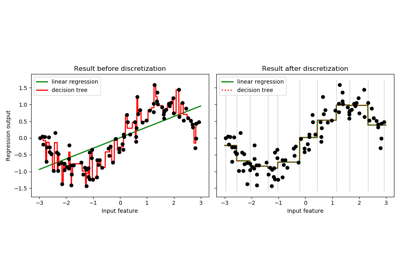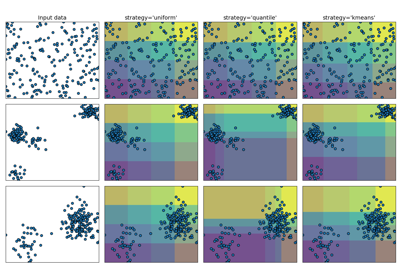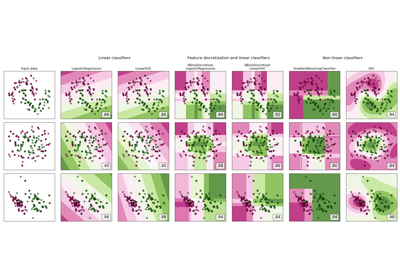# sklearn.preprocessing.KBinsDiscretizer¶

class sklearn.preprocessing.KBinsDiscretizer(n_bins=5, encode='onehot', strategy='quantile')[source]

Bin continuous data into intervals.

Read more in the User Guide.

Parameters: n_bins : int or array-like, shape (n_features,) (default=5) The number of bins to produce. Raises ValueError if n_bins < 2. encode : {‘onehot’, ‘onehot-dense’, ‘ordinal’}, (default=’onehot’) Method used to encode the transformed result. onehot Encode the transformed result with one-hot encoding and return a sparse matrix. Ignored features are always stacked to the right. onehot-dense Encode the transformed result with one-hot encoding and return a dense array. Ignored features are always stacked to the right. ordinal Return the bin identifier encoded as an integer value. strategy : {‘uniform’, ‘quantile’, ‘kmeans’}, (default=’quantile’) Strategy used to define the widths of the bins. uniform All bins in each feature have identical widths. quantile All bins in each feature have the same number of points. kmeans Values in each bin have the same nearest center of a 1D k-means cluster. n_bins_ : int array, shape (n_features,) Number of bins per feature. Bins whose width are too small (i.e., <= 1e-8) are removed with a warning. bin_edges_ : array of arrays, shape (n_features, ) The edges of each bin. Contain arrays of varying shapes (n_bins_, ) Ignored features will have empty arrays.

sklearn.preprocessing.Binarizer
class used to bin values as 0 or 1 based on a parameter threshold.

Notes

In bin edges for feature i, the first and last values are used only for inverse_transform. During transform, bin edges are extended to:

np.concatenate([-np.inf, bin_edges_[i][1:-1], np.inf])


You can combine KBinsDiscretizer with sklearn.compose.ColumnTransformer if you only want to preprocess part of the features.

KBinsDiscretizer might produce constant features (e.g., when encode = 'onehot' and certain bins do not contain any data). These features can be removed with feature selection algorithms (e.g., sklearn.feature_selection.VarianceThreshold).

Examples

>>> X = [[-2, 1, -4,   -1],
...      [-1, 2, -3, -0.5],
...      [ 0, 3, -2,  0.5],
...      [ 1, 4, -1,    2]]
>>> est = KBinsDiscretizer(n_bins=3, encode='ordinal', strategy='uniform')
>>> est.fit(X)
KBinsDiscretizer(...)
>>> Xt = est.transform(X)
>>> Xt
array([[ 0., 0., 0., 0.],
[ 1., 1., 1., 0.],
[ 2., 2., 2., 1.],
[ 2., 2., 2., 2.]])


Sometimes it may be useful to convert the data back into the original feature space. The inverse_transform function converts the binned data into the original feature space. Each value will be equal to the mean of the two bin edges.

>>> est.bin_edges_
array([-2., -1.,  0.,  1.])
>>> est.inverse_transform(Xt)
array([[-1.5,  1.5, -3.5, -0.5],
[-0.5,  2.5, -2.5, -0.5],
[ 0.5,  3.5, -1.5,  0.5],
[ 0.5,  3.5, -1.5,  1.5]])


Methods

 fit(X[, y]) Fits the estimator. fit_transform(X[, y]) Fit to data, then transform it. get_params([deep]) Get parameters for this estimator. inverse_transform(Xt) Transforms discretized data back to original feature space. set_params(**params) Set the parameters of this estimator. transform(X) Discretizes the data.
__init__(n_bins=5, encode='onehot', strategy='quantile')[source]
fit(X, y=None)[source]

Fits the estimator.

Parameters: X : numeric array-like, shape (n_samples, n_features) Data to be discretized. y : ignored self
fit_transform(X, y=None, **fit_params)[source]

Fit to data, then transform it.

Fits transformer to X and y with optional parameters fit_params and returns a transformed version of X.

Parameters: X : numpy array of shape [n_samples, n_features] Training set. y : numpy array of shape [n_samples] Target values. X_new : numpy array of shape [n_samples, n_features_new] Transformed array.
get_params(deep=True)[source]

Get parameters for this estimator.

Parameters: deep : boolean, optional If True, will return the parameters for this estimator and contained subobjects that are estimators. params : mapping of string to any Parameter names mapped to their values.
inverse_transform(Xt)[source]

Transforms discretized data back to original feature space.

Note that this function does not regenerate the original data due to discretization rounding.

Parameters: Xt : numeric array-like, shape (n_sample, n_features) Transformed data in the binned space. Xinv : numeric array-like Data in the original feature space.
set_params(**params)[source]

Set the parameters of this estimator.

The method works on simple estimators as well as on nested objects (such as pipelines). The latter have parameters of the form <component>__<parameter> so that it’s possible to update each component of a nested object.

Returns: self
transform(X)[source]

Discretizes the data.

Parameters: X : numeric array-like, shape (n_samples, n_features) Data to be discretized. Xt : numeric array-like or sparse matrix Data in the binned space.

## Examples using sklearn.preprocessing.KBinsDiscretizer¶Using KBinsDiscretizer to discretize continuous featuresDemonstrating the different strategies of KBinsDiscretizerFeature discretization